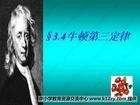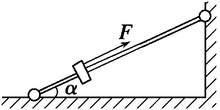Language :
 Search
 Picture : Type :[|jpg|gif|jpeg|png|] Byte :[＜2000KB] Language : 中文(简体) 中文(繁體) English 日本 한국어 Deutsch Française Ελληνικά Россию svenska Nederlandse Polska Український dansk български Italiano Icelandic român suomen kieli Galicia Türk Pilipino Català český hrvatski Latvijas Lietuvos македонски norsk språk Српски језик slovenský jazyk slovenščina Magyar nyelv فارسی Português ไทย Español Bahasa Indonesia Ngôn ngữ Việt Nam العربية Gaeilge shqiptar eesti Беларускія Die Boole-taal (Afrikaans) Malti Melayu lugha ya Kiswahili Cymraeg עברית שפה ייִדיש हिन्दी esperanto bosanski اردو زبان Azərbaycan ქართული Kreyòl ayisyen Euskal հայերեն ગુજરાતી ಕನ್ನಡ latin ພາສາລາວ বাংলা ভাষা తెలుగు தமிழ் மொழி ខ្មែរ | Check code :[Visitor (120.204.*.*)]answers [Chinese ] Time :2021-09-29Newton's Third Law (Force and Reaction Force Law)contentThe forces of action and reaction between two objects are equal in size and opposite in the same straight line. (See Newton's Third Law of Motion)Expression: F-F'The third law(F indicates force, F' indicates reaction force, and a negative sign indicates reaction force F' in the opposite direction to force F)illustrateTo change the motion of an object, other objects must interact with it. The interaction between objects is reflected by force. And pointed out that the role of force is mutual, there must be force reaction. They act on the same straight line, equal in size, in the opposite direction.note1.(1) The effect of force is mutual. Appears at the same time and disappears at the same time.(2) The interaction force must be a force of the same nature(3) The force and reaction force act on two objects, and the effects produced cannot be offset by each other.(4) The force can also be called the reaction force, but the choice of reference is different(5) Force and reaction force because the point of action is not on the same object, so can not seek joint force2. The difference between the interaction force and the equilibrium force(1) The interaction force is a force of equal size, opposite direction, acting on two objects, and in the same straight line; The nature of the two forces is the same.(2) The equilibrium force is two forces acting on the same object, the same size, opposite direction, and acting in the same straight line. The nature of the two forces can be different.(3) Two forces that are balanced can exist alone, but the interaction forces exist at the same time and disappear at the same timeFor example, an object is placed on a table, and for the gravity and support forces that an object is subjected to, the two are balanced forces, the support force disappears after the object is removed, and gravity still exists.And the object on the table, the object is subjected to the support force and the pressure on the table table, the two are a pair of forces and reaction force. Both disappear when the object is taken away.
 [Visitor (120.204.*.*)]answers [Chinese ] Time :2021-09-29Newton's Second Law (The Law of Acceleration)contentThe acceleration of an object is proportional to the force of the outer force of the object, inversely proportional to the mass of the object, and the direction of acceleration is the same as the direction of the force.formula:F-in-ma (units: N (nx) or kgm per second) N s (kg×m) / (s×s)Newton's original formula: F-mv/tUnder the action of object F with a momentum of p, the rate of change of momentum over time is equal to the force of the outer force acting on the object. , in the combined external force forIn layman's terms, the derivative of a function with t as the argument and p as the dependent variable is the combined external force that the point is subjected to.That is: F-dpd/t-d (mv)/dt (d is not delta( t), but differential. But in the general problem of secondary school study, the two can not make a difference)And when an object moves at low speed, at a much lower speed than the speed of light, the mass of the object is a constant that does not depend on speed, so there isF=m(dv/dt)=maThis is also called the momentum theorem. In relativity, F-ma is not valid because mass changes with speed, and F-d (mv)/dt is still used.From the experiment can be obtained in the acceleration of a certain situation F and m proportional, in the mass of a certain situation F and a is proportional(F is only established if F is in N, m in kg, and a is in m/s2)A few points:The second law(1) Newton's second law is the transient action law of force. Forces and accelerations are produced at the same time, change at the same time, and disappear at the same time.(2) F-ma is a vector equation, the application should specify the positive direction, where the same force or acceleration with the positive direction are positive, and vice versa to take negative values, generally take the direction of acceleration is positive direction.(3) According to the principle of independent action of force, when dealing with the motion of an object in a plane with Newton's second law, the orthogonal decomposition of the forces subjected to the object can be applied to the component form of Newton's second law in two directions perpendicular to each other: Fx-max, Fy-may column equation.(4) Newton's second law applies only to the movement of mass.Six natures(1) Causality: Force is the cause of acceleration.(2) Hompathy: F, m, a corresponds to the same object.(3) Vector: Force and acceleration are vectors, and the direction of acceleration of an object is determined by the direction of the external forces that the object is subjected to. Newton's second law mathematical expression ∑ F , the equal sign not only indicates that the values on the left and right are equal, but also indicates the same direction, i.e. the acceleration direction of the object is the same as the direction of the combined external force.(4) Transient: when the external force of an object (mass must) undergo a sudden change, the size and direction of acceleration determined by force should also be mutated at the same time; When the combined external force is zero, the acceleration is zero at the same time, and the acceleration and the combined external force maintain a one-to-one correspondence. Newton's second law is a transient corresponding law, indicating the instantaneous effect of force.(5) Relativity: There is a coordinate system in nature in which the motion or stationary state of a straight line at a constant speed is maintained when an object is not forced, such a coordinate system is called an inertial frame of reference. The ground and objects that are stationary relative to the ground or move in a constant linear line can be regarded as inertial reference systems, and Newton's law is only established in inertial frames.(6) Independence: each force acting on an object can independently produce an acceleration, the acceleration of each force produces a vector and is equal to the acceleration generated by the combined external force.Scope of application(1) Only for objects with low speed motion (lower than the speed of light).(2) Only applies to macro-objects, Newton's second law does not apply to microetoms.(3) The reference system shall be the inertial system.
 [Visitor (120.204.*.*)]answers [Chinese ] Time :2021-09-29Newton's First Law (The Law of Inertia)contentExpression 1: When an object is not subjected to external forces or equilibrium forces (Fnet=0), it always remains stationary or in a constant linear motion until the external forces acting on it force it to change this state.The original static object has the nature of maintaining stillness, the original moving object has the nature of keeping motion, so we call the object has the nature of keeping the motion state unchanged called inertia. All objects have inertia, which is the physical property of objects. So this law is also called the law of inertia.Expression 2: When the mass is far enough away from the other mass, the mass is moved in a constant linear line or remains stationary.That is, mass is a measure of inertia size.Inertia size is only related to mass, not speed and roughness of the contact surface.The greater the mass, the greater the work to overcome inertia; The smaller the mass, the smaller the effort to overcome inertia.Force is not the cause of the motion of an object, but the cause of changing the motion of an object.